# Understanding STAR-DELTA Starter !

## What is Star Delta Starter ?

A star delta starter is the most commonly used method for the starting of a 3 phase induction motor. In star delta starting an induction motor is connected in through a star connection throughout the starting period. Then once the motor reaches the required speed, the motor is connected in through a delta connection.

A star delta starter will start a motor with a star connected stator winding. When motor reaches about 80% of its full load speed, it will begin to run in a delta connected stator winding.A star delta starter is a type of reduced voltage starter. We use it to reduce the starting current of the motor without using any external device or apparatus. This is a big advantage of a star delta starter, as it typically has around 1/3 of the inrush current compared to a DOL starter.
The starter mainly consists of a TPDP switch which stands for Tripple Pole Double Throw switch. This switch changes stator winding from star to delta. During starting condition stator winding is connected in the form of a star. Now we shall see how a star delta starter reduces the starting current of a three-phase induction motor.

For that let us consider,

VL = Supply Line Voltage, ILS = Supply Line Current and, IPS = Winding Current per Phase and Z = Impedance per phase winding at stand still condition.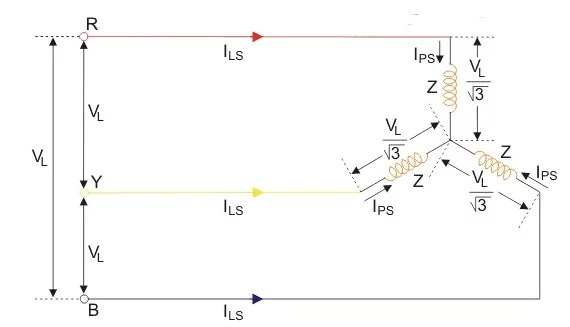As the winding is star connected, the winding current per phase (IPS) equals to supply line current (ILS).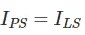As the winding is star connected, the voltage across each phase of the winding isHence, the winding current per phase is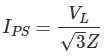Since here, the winding current per phase (IPS) equals to the supply line current (ILS), we can write,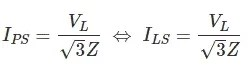Now, let us consider the situation where the motor gets started with delta connected stator winding from same three phase supply points,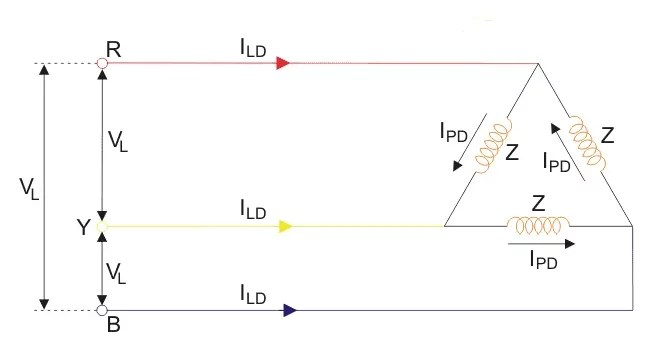Here, ILD = Supply Line Current and, IPD = Winding Current per Phase and Z = Impedance per phase winding at stand still condition.
As the winding is delta connected, supply line current (ILD) is root three times of the winding current per phase (IPD)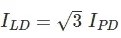As the winding is delta connected, the voltage across each phase of the winding is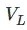Hence, the winding current per phase is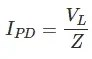Now, we can write,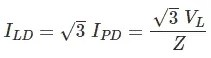Now, by comparing supply line currents drawn by an induction motor with star and delta connected winding, we getThus we can say that the starting current from the mains in case of star delta is one-third of direct switching in the delta. Again, we know that the starting torque of an induction motor is proportional to the square of the voltage applied to the winding per phase.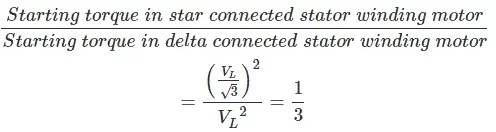The equation shows that star delta starter reduces the starting torque to one-third of that produced by DOL starter. The star-delta starter is equivalent to an autotransformer with a 57.7% tapping.

You might have seen that in order to start a high power rating induction motor, a starting technique called star-delta is used. We will understand why a star-delta starter is needed, and how it is accomplished practically with help of animation.

## Like it? Share with your friends!Dislike
594
Dislikelove
2973
loveomg
2378
omgscary
2081
scarywtf
1189
wtf